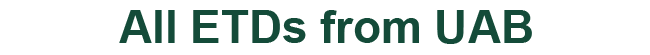### Expansion in Eigenfunctions for Systems of Ordinary Differential Equations Without the Unique Continuation Property

Rudi Weikard

David Cruz-Uribe

John Johnstone

Claudio Morales

Atanas Stefanov

Dissertation

2023

#### Degree Name by School

Doctor of Philosophy (PhD) College of Arts and Sciences

#### Abstract

In this document we consider differential equations where the coefficients are matrices whose entries are distributions of order zero satisfying certain criteria. The derivative which occurs in the equation is the distributional derivative. Chapter 1, sections 1-7 contain information necessary to understand for the reading of Chapters 2 and 3. Section 8 gives a brief introduction to what has been done so far and our goal. Thereafter, in Chapter 2, it is shown that self-adjoint restrictions of the maximal relation to the differential equation are still given by boundary conditions. With that done, given a self-adjoint restriction of the maximal relation, it is shown that the resolvent operator at a fixed point in the resolvent set is an integral operator with an integral kernel, i.e. a Green’s function exists. In Chapter 3, we investigate the form of this Green’s function under certain further assumptions on the coefficients of the equation and establish a generalized Fourier transform. One deviation from the classical case is that our Fourier transform is not injective, but many properties do carry over to this setting. In the course of establishing this generalized Fourier transform, interesting facts as well as a Fatou-type convergence were found which are also recorded.

COinS